You can Download Samacheer Kalvi 9th Maths Book Solutions Guide Pdf, Tamilnadu State Board help you to revise the complete Syllabus and score more marks in your examinations.

## Tamilnadu Samacheer Kalvi 9th Maths Solutions Chapter 2 Real Numbers Ex 2.9

Multiple Choice Questions :
Question 1.
If n is a natural number then $$\sqrt{n}$$ is
(1) always a natural number
(2) always an irrational number
(3) always a rational number
(4) may be rational or irrational
Solution:
(4) may be rational or irrational

Question 2.
Which of the following is not true?
(1) Every rational number is a real number.
(2) Every integer is a rational number.
(3) Every real number is an irrational number.
(4) Every natural number is a whole number.
Solution:
(3) Every real number is an irrational number
Hint:
Real numbers contain rationals and irrationals.

Question 3.
Which one of the following, regarding sum of two irrational numbers, is true?
(1) always an irrational number
(2) may be a rational or irrational number.
(3) always a rational number
(4) always an integer.
Solution:
(2) may be a rational or irrational number

Question 4.
Which one of the following has a terminating decimal expansion?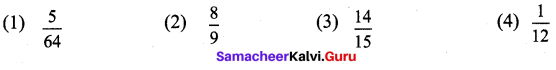Solution:
(1) $$\frac { 5 }{ 64 }$$
Hint:
$$\frac { 5 }{ 64 }$$ = $$\frac{5}{2^{6}}$$Question 5.
Which one of the following is an irrational number?
(1) $$\sqrt { 25 }$$
(2) $$\sqrt { \frac { 9 }{ 4 } }$$
(3) $$\frac { 7 }{ 11 }$$
(4) π
Solution:
(4) π
Hint:
π represents a irrational number

Question 6.
An irrational number between 2 and 2.5 is
(1) $$\sqrt { 11 }$$
(2) $$\sqrt { 5 }$$
(3) $$\sqrt { 2.5 }$$
(4) $$\sqrt { 8 }$$
Solution:
(2) $$\sqrt { 5 }$$
Hint:
22 = 4 and 2.52 = 6.25

Question 7.
The smallest rational number by which – should be multiplied so that its decimal expansion terminates after one place of decimal is
(1) $$\frac { 1 }{ 10 }$$
(2) $$\frac { 3 }{ 10 }$$
(3) 3
(4) 30
Solution:
(2) $$\frac { 3 }{ 10 }$$
Hint:
$$\frac { 3 }{ 10 }$$ is the small number.

Question 8.
If $$\frac { 1 }{ 7 }$$ = $$0.\overline { 142857 }$$ then the value of $$\frac { 5 }{ 7 }$$ is
(1) $$0.\overline { 142857 }$$
(2) $$0.\overline { 714285 }$$
(3) $$1.\overline { 571428 }$$
(4) 0.714285
Solution:
(2) $$0.\overline { 714285 }$$
Hint:
5 × $$\frac { 1 }{ 7 }$$ = 5 × $$0.\overline { 142857 }$$ = $$0.\overline { 714285 }$$Question 9.
Find the odd one out of the following.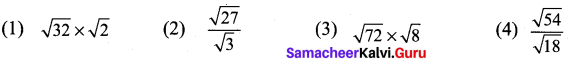Solution:
(4) $$\frac{\sqrt{54}}{\sqrt{18}}$$
Hint:
$$\sqrt { 72 }$$ × $$\sqrt { 8 }$$ = $$\sqrt { 9\times8 }$$ × $$\sqrt { 8 }$$ = 3 × 8 = 24

Question 10.
$$0.\overline { 34 }$$ + $$0.3\overline { 4 }$$ =
(1) $$0.6\overline { 87 }$$
(2) $$0.\overline { 68 }$$
(3) $$0.6\overline { 8 }$$
(4) $$0.68\overline { 7 }$$
Solution:
(1) $$0.6\overline { 87 }$$
Hint:
0.343434 … + 0.344444 … = $$0.6\overline { 87 }$$

Question 11.
Which of the following statement is false?
(1) The square root of 25 is 5 or -5
(2) $$\sqrt { 25 }$$ = 5
(3) –$$\sqrt { 25 }$$ = -5
(4) $$\sqrt { 25 }$$= ±5
Solution:
(4) $$\sqrt { 25 }$$ = ±5

Question 12.
Which one of the following is not a rational number?
(1) $$\sqrt { \frac { 8 }{ 18 } }$$
(2) $$\frac { 7 }{ 3 }$$
(3) $$\sqrt { 0.01 }$$
(4) $$\sqrt { 13 }$$
Solution:
(4) $$\sqrt { 13 }$$
Hint:
(1) $$\sqrt { \frac { 8 }{ 18 } }$$ = $$\sqrt { \frac { 4 }{ 9 } }$$ = $$\frac { 2 }{ 3 }$$ is a arational number
(2) $$\frac { 7 }{ 3 }$$ is a rational number
(3) $$\sqrt { 0.01 }$$ = $$\sqrt { \frac { 1 }{ 100 } }$$ = $$\frac { 2 }{ 3 }$$ is a rational number
(4) $$\sqrt { 13 }$$ is a rational numberQuestion 13.
$$\sqrt { 27 }$$ + $$\sqrt { 12 }$$ =
(1) $$\sqrt { 39 }$$
(2) $$5\sqrt { 6 }$$
(3) $$5\sqrt { 3 }$$
(4) $$3\sqrt { 5 }$$
Solution:
(3) $$5\sqrt { 3 }$$
Hint:
$$\sqrt { 27 }$$ + $$\sqrt { 12 }$$ = $$\sqrt{9 \times 3}+\sqrt{4 \times 3}=3 \sqrt{3}+2 \sqrt{3}=5 \sqrt{3}$$

Question 14.
if $$\sqrt { 80 }$$ = k$$\sqrt { 5 }$$, then k =
(1) 2
(2) 4
(3) 8
(4) 16
Solution:
(2) 4
Hint: $$\sqrt { 80 }$$ = $$\sqrt{16 \times 5}=4 \sqrt{5}=k \sqrt{5}$$ ⇒ k = 4

Question 15.
$$4 \sqrt{7} \times 2 \sqrt{3}$$ =
(1) 6$$\sqrt{10}$$
(2) 8$$\sqrt{21}$$
(3) 8$$\sqrt{10}$$
(4) 6$$\sqrt{21}$$
Solution:
(2) 8$$\sqrt{21}$$
Hint:
$$4 \sqrt{7} \times 2 \sqrt{3}$$ = $$8\times\sqrt{7 \times 3}$$ = 8$$\sqrt{21}$$

Question 16.
When written with a rational denominator, the expression $$\frac{2 \sqrt{3}}{3 \sqrt{2}}$$ can be simplified as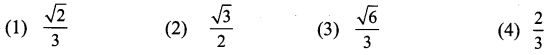Solution:
(3) $$\frac{\sqrt{6}}{3}$$
Hint:
$$\frac{2 \sqrt{3}}{3 \sqrt{2}}=\frac{2 \sqrt{3}}{3 \sqrt{2}} \times \frac{\sqrt{2}}{\sqrt{2}}=\frac{2 \sqrt{6}}{3 \times 2}=\frac{2 \sqrt{6}}{63}$$

Question 17.
When (2$$\sqrt{5}$$ – $$\sqrt{2}$$)2 is simplified, we get
(1) 4$$\sqrt{5}$$ + 2$$\sqrt{2}$$
(2) 22 – 4$$\sqrt{10}$$
(3) 8 – 4$$\sqrt{10}$$
(4) 2$$\sqrt{10}$$ – 2
Solution:
(2) 22 – 4$$\sqrt{10}$$
Hint:
(2$$\sqrt{5}$$ – $$\sqrt{2}$$)2 = (2$$\sqrt{5}$$)2 – 2 × 2$$\sqrt{5}$$ × $$\sqrt{2}$$ + $$\sqrt{2^{2}}$$
= 4 × 5 – 4$$\sqrt{10}$$ + 2 = 22 – 4$$\sqrt{10}$$Question 18.
(0.000729)$$\frac{-3}{4}$$ × (0.09)$$\frac{-3}{4}$$ = ____.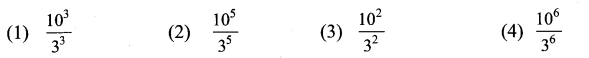Solution:
(4) $$\frac{10^{6}}{3^{6}}$$
Hint :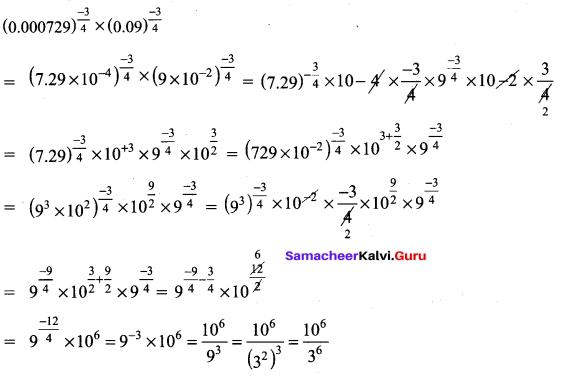Question 19.
If $$\sqrt{9^{x}}=\sqrt{9^{2}}$$ , than x = ___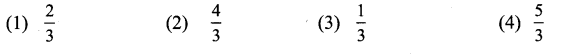Solution:
(2) $$\frac { 4 }{ 3 }$$
Hint: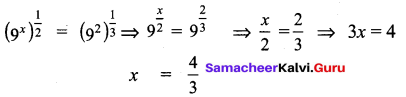Question 20.
The length and breadth of a rectangular plot are 5 x 105 and 4 x 104 metres respectively. Its area is .
(1) 9 × 101 m2
(2) 9 × 109 m2
(3) 2 × 1010 m2
(4) 20 × 1020 m2
Solution:
(3) 2 × 1010 m2
Hint:
l = 5 × 105 metres; b = 4 × 104 metres
∴ Area = l × b = 5 x 105 × 4 × 104
= 20 × 105+4= 20 × 109= 2.0 × 101 × 109 = 2 × 1010m2